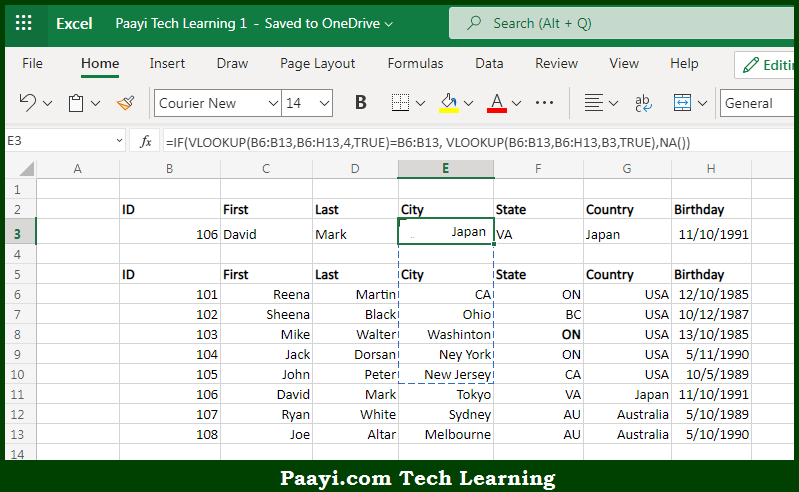# Learn How to Faster VLOOKUP with 2 VLOOKUPS in Microsoft Excel

Written by | 0 Comments | 330 Views

In this article, you will learn how to evaluate things based on the LOOKUP function in Microsoft Excel using a single/combination(s) of functions. You will also know to Faster VLOOKUP with 2 VLOOKUPS and see the generic formula.

Learn How to Faster VLOOKUP with 2 VLOOKUPS in Microsoft Excel

The main purpose of this formula is to make VLOOKUP lightning fast by using two VLOOKUPS. Here we will learn how to faster VLOOKUP with two VLOOKUPS in the given data range in the workbook in Microsoft Excel. That implies, with the help of a formula based on the VLOOKPUS functions you can able to make VLOOKUP lightning fast by using two VLOOKUPS, with large sets of data, exact match VLOOKUP can be painfully slow. So, with the help of this formula, you can able to faster VLOOKUP with two VLOOKUPS in the given data range in the workbook in Microsoft Excel.

General Formula to Faster VLOOKUP with 2 VLOOKUPS

=IF(VLOOKUP(id,data,1,TRUE)=id, VLOOKUP(id,data,col,TRUE), NA())

The Explanation to Faster VLOOKUP with 2 VLOOKUPSSo we know that with the help of the given formula above you can able to make VLOOKUP lightning fast by using two VLOOKUPS. Here we will learn how to faster VLOOKUP with two VLOOKUPS in the given data range in the workbook in Microsoft Excel. As we know that If you have a smaller set of data, this approach is overkill. Only use it with large data sets when speed really counts. You must sort the data by lookup the value in order for this trick to work. This example uses named ranges. If you don't want to use named ranges use absolute references instead. So now you have learned how to faster VLOOKUP with two VLOOKUPS in the given data range in the workbook in Microsoft Excel.